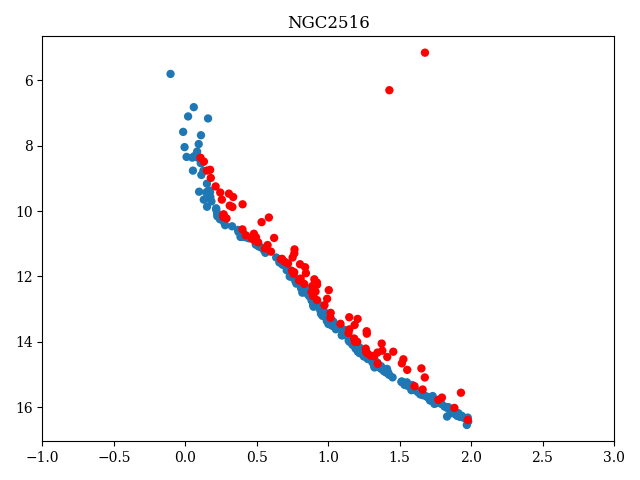# 10.6.2 Photometry: BP, RP magnitudes

We assess the quality of the $G_{\rm BP}$, $G_{\rm RP}$ photometry by estimating the width of the sequence in the CMD of clusters with secure membership. A downside of having to rely on secure membership means that it is difficult to provide diagnostics for stars fainter than $G\sim 18$, as the astrometry (and thus our ability to tell cluster stars apart from field stars) strongly decreases in quality at that magnitude.

To avoid differential reddening artificially increasing the width of the sequence, we limited our diagnostics to clusters with little extinction variations across the field (e.g., Figure 10.47). We use a LOWESS fitting to follow the sequence, and remove binary stars and stars deviating from the main sequence by clipping out sources with $G_{\rm BP}$ fluxes two error bars lower and $G_{\rm RP}$ fluxes higher than the fitted relation.Figure 10.47: CMD of NGC 2516, in red the stars that are discarded.

We obtain very clean sequences for all the OCs. An illustration is shown Figure 10.48 for NGC 2682. The typical dispersion in both $G_{\rm BP}$ and $G_{\rm RP}$ is of the order of 0.02 mag. Scaling the difference to the fitted relation by the individual error bars of each star we find a dispersion of, on the average, 1.7 for $G_{\rm BP}$ and 2 for $G_{\rm RP}$, suggesting that the errors on magnitudes in both filters tend to be underestimated. By limiting the sample to stars well below the turn off, those numbers decrease to 1.3 and 1.5, respectively. The turnoff region is affected by evolutive effects and might have the presence of rapidly rotating stars.

In all cases, the dispersion in units of nominal errors always appears slightly larger in the $G_{\rm RP}$ filter than in $G_{\rm BP}$.Figure 10.48: The only visible points are the cluster members, after removing binary stars. Top rows: LOWESS fit of the GBP (resp. GRP) versus G relation. Middle rows: residuals of the fit. Bottom: residuals scaled by individual error bars. Right: CMD (from Arenou et al. 2018).